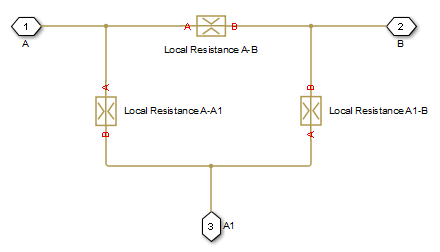# T-junction

(To be removed) Hydraulic resistance of T-junction in pipe

The Hydraulics (Isothermal) library will be removed in a future release. Use the Isothermal Liquid library instead. (since R2020a)

## Library

Local Hydraulic Resistances

•## Description

The T-junction block represents a T-junction (wye connection) consisting, in general, of a main run and a branch merging to the main run. The junction as a hydraulic resistance is built of three Local Resistance blocks, as shown in the following diagram.To specify pressure loss for all possible flow directions, you have to provide six pressure loss coefficients. The flow regime is checked in the underlying Local Resistance blocks by comparing the Reynolds number to the specified critical Reynolds number value, and depending on the result, the appropriate formula for pressure loss computation is used. For more information, see the reference documentation for the Local Resistance block.

The block positive direction is from port A to port B, from port A to port A1, and from port A1 to port B.

## Basic Assumptions and Limitations

• Fluid inertia is not taken into account.

## Parameters

### Geometry Tab

Main pipe diameter

The internal pipe diameter of the main run. The default value is `0.01` m.

Branch pipe diameter

The internal pipe diameter of the branch. The default value is `0.01` m.

Laminar transition specification

Select how the block transitions between the laminar and turbulent regimes:

• `Pressure ratio` — The transition from laminar to turbulent regime is smooth and depends on the value of the Laminar flow pressure ratio parameter. This method provides better simulation robustness.

• `Reynolds number` — The transition from laminar to turbulent regime is assumed to take place when the Reynolds number reaches the value specified by the Critical Reynolds number parameter.

Laminar flow pressure ratio

Pressure ratio at which the flow transitions between laminar and turbulent regimes. The default value is `0.999`. This parameter is visible only if the Laminar transition specification parameter is set to ```Pressure ratio```.

Critical Reynolds number

The maximum Reynolds number for laminar flow. The value of the parameter depends on the orifice geometrical profile. You can find recommendations on the parameter value in hydraulics textbooks. The default value is `120`. This parameter is visible only if the Laminar transition specification parameter is set to `Reynolds number`.

### Pressure Loss Tab

A-B pressure loss coefficient

The pressure loss coefficient between ports A and B when fluid flows in the direction from A to B. The default value is `1.12`.

B-A pressure loss coefficient

The pressure loss coefficient between ports A and B when fluid flows in the direction from B to A. The default value is `1.12`.

A-A1 pressure loss coefficient

The pressure loss coefficient between ports A and A1 when fluid flows in the direction from A to A1. The default value is `1.36`.

A1-A pressure loss coefficient

The pressure loss coefficient between ports A and A1 when fluid flows in the direction from A1 to A. The default value is `1.65`.

A1-B pressure loss coefficient

The pressure loss coefficient between ports A1 and B when fluid flows in the direction from A1 to B. The default value is `1.6`.

B-A1 pressure loss coefficient

The pressure loss coefficient between ports A1 and B when fluid flows in the direction from B to A1. The default value is `1.8`.

## Global Parameters

Parameters determined by the type of working fluid:

• Fluid density

• Fluid kinematic viscosity

Use the Hydraulic Fluid block or the Custom Hydraulic Fluid block to specify the fluid properties.

## Ports

The block has the following ports:

`A`

Hydraulic conserving port associated with the main run inlet.

`B`

Hydraulic conserving port associated with the main run outlet.

`A1`

Hydraulic conserving port associated with the branch inlet.

## Version History

Introduced in R2006b

expand all